##### 10 - Relativity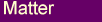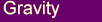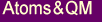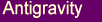Given the important role that relativity has played in the rejection of the aether concept by mainstream science we examine here in some detail relativity from an aether perspective.
We argue that many of the contradictions of standard relativity, such as the twin paradox, can only be satisfactorily resolved by assuming a medium that transmits light.

#### What is Wrong with Relativity?

When one looks into the matter carefully one discovers that there many problems with Einstein's theory of relativity, both at the conceptual level and experimentally.
In an outstanding piece of work, Milan Pavlovic has carefully examined Einstein's original special relativity papers and found them to contain many inconsistencies and questionable assumptions .
Further he looked at the experimental evidence used to justify the special theory of relativity, such as the Michelson-Morley experiment, the Doppler effect for light, Fizeau's light through water test, aberration of starlight. He showed that most of these could be understood in non-relativistic terms or with the assumption that the earth entrains the aether.
Other objections to the theory have been raised by various scientists over the years, see for example Burniston Brown's classic article  that takes a critical look at the special and general theories of relativity, as well as the Marcus Coleman article  that catalogs objections by well known physicists and mathematicians. See also the article by John A Hickli .
Those who have looked into the matter  have documented more than 3000 articles published in scientific literature that criticize the special theory of relativity. Articles which have been largely ignored or conveniently swept under the carpet.
Experimentally too there are disagreements with Einstein's relativity.
One of the postulates on which the special theory of relativity is based states that the speed of light is constant for any observer. However a number of experiments contradict this, for example Dayton Miller's  and Yuri Galaev's  Michelson-Morley type experiments that produced a definite positive result under certain conditions. If the postulate was correct no Michelson-Morley type experiment would produce a positive result.
Our Aether page discusses the 'null' result of the Michelson-Morley experiment and how it can be explained within the context of an aether.
As well there is experimental evidence emerging that the speed of light might not be so constant after all, .
Also the experiments of Eugene Podkletnov and Giovanni Modanese suggest that it is possible to transfer a signal faster than the speed of light. They used a high voltage discharge mechanism to generate what they refer to as a gravity wave impulse . This impulse was found to travel through thick metal and brick walls, and was able to affect objects a long distance away from the source. More important to the present discussion is the fact that they measured the beam speed to be more than 60 times the speed of light!
Chinese researchers have also shown experimentally that the quantum entanglement's action at a distance acts at many times the speed of light .
See also the following link  for a clear and concise description of the conceptual difficulties inherent in special relativity. This site also provides a good description of several experiments that are relevant to the aether versus special relativity debate.
Other evidence exists that supports the existence of a medium that transmits light, see for example Webster Kehr's  work.

However, in the author's opinion, it is the unresolved contradictions around the twin paradox and time dilation that provide the most compelling argument against special relativity. They highlight contradictions that make the precepts of special relativity untenable.
Relativity predicts that time slows down for a moving object relative to a stationary observer.
That is, if a moving clock is brought back to its starting position it should show a difference in the time registered compared to a stationery observer.
The slowing down of travelling clocks has been confirmed by use of atomic clocks, etc.
One cannot really explain it on the basis of the finite speed of light, or issues of simultaneity, etc, because the traveling clock could easily retrace its steps to end up in its starting position without any communication or interaction between the two clocks.
And given that special relativity postulates that all reference frames traveling at constant speed are equivalent as far as the laws of physics are concerned, there is no real reason why the two clocks should move forward at different rates.
There has to be something different about the traveling clock that makes it 'tick' more slowly.
One might suppose for example, as standard theory does, that the explanation lies in the fact that the traveling clock has to first accelerate to reach a certain speed and then decelerate to turn around and go back to the starting point, and it is this acceleration which 'causes' the slowing down of the traveling clock.
However this is inadequate as the total time difference is dependent on how long the clock moves at constant speed and not on how that speed was reached which is dependant on the acceleration. In other words, we could have two moving clocks each of which receives the same acceleration and reaches the same velocity but where one travels at that constant velocity for much longer than the other before returning. This gives rise to the situation where the two clocks would show different times relative to the stay at home clock even though they underwent exactly the same acceleration.
Is time dilation caused by acceleration or relative motion? If the time difference was due to the acceleration the relativistic formula should be expressed as a function of the acceleration and not the velocity. The relativistic time dilation formula being: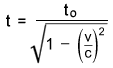We can also consider a variation of the twin paradox thought experiment. Suppose we have two identical twins, having identical rockets, who both accelerate in opposite directions for the same amount of time and then return back to their original positions. Special relativity predicts that each twin will see his brother as being younger.
The standard twin paradox is explained away by pointing to the fact that one twin is accelerating and the other is not. However in this example both twins experience the same accelerations and velocities - the paradox still remains.
When the equations describing time dilation have no connection with its supposed cause (acceleration) is it any wonder that we encounter paradoxical situations?

Some people have argued that we cannot use special relativity in the above examples because special relativity only applies to reference frames that are moving at constant velocity. The above examples involve acceleration so we should, the argument goes, use general relativity instead.
However it is not too difficult to come up with time dilation examples where the acceleration is taken out of the picture while the contradiction remains  .
One of the best examples of this is the time dilation phenomenon observed with high speed muon particles generated in the upper atmosphere. It is observed that high energy collisions between cosmic-ray particles and nuclei in the atmosphere generate muons, some of which travel towards the earth at close to the speed of light.
Normally muons are unstable and decay with a half life in the micro second range. However, because these are moving at a high velocity their half life increases in accord with the time dilation formula so that a lot more reach the earth than would be expected without time dilation. Experiment has confirmed that the number that reach the earth is in agreement with the time dilation formula.
Here we have an example of real time dilation with no acceleration effects. The muons don't decelerate and turn around in order to get back to their starting point. Note that the acceleration due to the gravitational attraction of the earth acting on the muons is so small in this case that it can for all practical purposes be ignored.
This example effectively proves that real time dilation is a velocity phenomenon.
And given that special relativity uses relative velocities we are back to the twin paradox scenario. The earth observer will see the muons moving towards the earth and will conclude that time will slow down for the muons relative to himself. However, according to the muon's perspective it is the earth observer that is moving towards it and therefore the observer's time should slow down relative to it.
So here we have a variation of the twin paradox where we cannot resort to acceleration to resolve the contradiction.
We should also add that standard theory tries to resolve this difficulty by resorting to length contraction arguments. However, if one looks at it carefully there are serious flaws in this argument, as there are in several aspects of special relativity.

In the next section we will look at time dilation from an aether perspective and show that the twin paradox can only be satisfactorily resolved by assuming a universal reference frame or a medium that transmits light.
The introduction of the aether brings back common sense to relativity, sparing us the need to perform mathematical contortions to make the speed of light be a constant for all observers.

#### Aether, Time Dilation and Special Relativity

Time is a consequence of the rate of change of processes which are used to measure it.
The most plausible explanation of time dilation is that the slowing down of the traveling clock is caused by it's interaction with its surroundings. In particular a slowing down of these internal processes with motion relative to an underlying medium such as the aether.
In a Caesium atomic clock for example it would be the emission frequency of a particular electron orbital in the Caesium atom.
We should really be talking about clock dilation rather than the dilation of time as is normally interpreted from special relativity. Time has no meaning divorced from some cyclic process whose rate of change is used to measure it.

In deriving the time dilation effects for an aether case we will consider the example that is often used in standard texts on relativity, the motion of a light clock.
The light clock in its most simplified form consists of a source and detector of light at one end of a rod and a mirror at the other, Fig 10.1(a).
The time it takes light to travel from the light source to the mirror and back again will be our unit of time or 'tick' of the clock.Fig 10.1 The light clock for two cases. (a) The clock is stationary relative to the aether.
(b) The clock moves to the right at velocity v relative to the aether.

We consider the two cases where the clock is stationary, Fig 10.1(a), and when it travels at velocity v relative to the aether, Fig 10.1(b), at right angles to the clock axis.
Given that light will always travel at speed c relative to the aether we have the following clock times (1 tick) for stationary and moving cases:where  L = length of the clock,   c = speed of lightwhere  H = diagonal length given byIt can be shown that the above values lead to the following relationship between the two clock times as a function of the velocity:The proportionality factor being given by the standard relativistic factor - a factor that has its origins in electromagnetism and was first derived by Lorentz.
So for a traveling light clock, time as measured by each tick of the clock would actually slow down compared to a stationary one. It is a real effect that follows directly from the constancy of light speed relative to the aether (independent of the speed of the source), and the fact that light has to travel paths of different length through the aether to complete one cycle of the clock (red lines in Fig 10.1).
The derivation of the formula is similar to the standard theory, the difference being that in the present example the speed of light is constant relative to the aether whereas in the standard theory it is assumed to be constant relative to any observer. This assumption leads to the contradictions inherent in the standard theory, such as the twin paradox example discussed in the previous section.
In the aether model we have no such contradictions. Time dilations only occur for the clock moving relative to the aether, so the twins will both agree on the differences registered by their light clocks.
In the case where both twins travel equal amounts relative to the aether they will both time dilate by the same amounts, so their clocks will show the same time, which would be different to a stationary clock, when they get back together.

The next question that arises is, will the time dilation derived above apply to any type of clock, any type of process? Will the traveling twin actually look younger, if enough time passes?
A clock can be anything that has a cyclic process.
Let us consider one of the simplest types of clock, a rotor consisting of a ball (green) at the end of a rigid arm that rotates at a constant angular speed, Fig 10.2.Fig 10.2 The rotor clock for two cases. (a) The clock is stationary relative to the aether.
(b) The clock moves to the right at velocity v relative to the aether.

Doing the same analysis as for the light clock, we have that the time it takes for the ball to complete one cycle of rotation will be our unit of time measurement.
Again we compare the case where the clock is stationary, Fig 10.2(a), with the one where the whole unit moves at velocity v relative to the aether, Fig 10.2(b). The rate of rotation is assumed to be the same for the two cases.
For ease of analysis we will assume that v in (b) above is the same as the rotational velocity of the ball (equivalent to cycloid motion), however a similar argument will apply for any v.
For the (a) case, one tick of the clock will correspond to the circumference, given by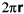, divided by the velocity of the rotating ball.
Given that the rotation rates are the same for both clocks, one tick for clock (b) will correspond to the case where the clock moves a distance, Fig 10.2(b), equivalent to the distance the rotating ball moves in the (a) case.
We can see from Fig 10.2(b) that in the equivalent time the rotating ball of (b) will have traveled a larger distance through the aether (red line) compared to (a) case.
Therefore, if we had an equivalent condition as for the light clock that the rotating ball moved at a constant velocity relative to the aether we would again have time dilation. The larger the value of v the greater the ball path compared to the stationary case (a).
However, clearly the ball will not travel at a constant velocity relative to the aether. The rigid arm will sometimes pull the ball through the aether faster and sometimes slower, depending on which part of the cycle it is on.
However we do not need to be concerned about these because the fact that the rigid arm rotates at the same rate for both clocks means that the clock cycles must also be the same for both. Therefore we can conclude that for a rigid rotating clock there will be no time dilation.

What happens if the arm is not rigid, such as for an electron spinning around an atom?
In that case there can certainly be a slowing down of the rotating electron, at least in parts of the cycle, leading to time dilation.
It is difficult to determine exactly what should happen as we do not know the details of what goes on inside an atom, however we would expect some type of disturbance of the electrons when an atom moves through the aether.
This would seem to be supported by experiments  which showed differences in the forces between strongly charged bodies when moving as compared to the stationary case (see also Magnetism page).
For a neutral object the effects of moving negative charges will be cancelled by the positive charges on a macro scale. However at an atomic scale the charges are separated and one would therefore expect small differences in the forces within an atom for moving objects compared to stationary ones.
Various time dilation experiments involving such things as atomic clocks, particle decay rates, etc, show that there is indeed time dilation at the atomic level. Furthermore, that atomic time dilation seems to follow the standard relativistic formula, as for the light clock discussed above.
Time dilation at the atomic level is in fact an additional argument for the existence of the aether.
From the rotor clock arguments above, if there were no aether or medium there would be nothing to affect the motion of the electrons and therefore the times for each cycle of the clock.
Given that special relativity postulates that all reference frames traveling at constant speed are equivalent as far as the laws of physics are concerned, without something like an aether there could not be any time dilation at the atomic level!

It also follows from the above argument that the reverse situation of a stationary object and a moving aether should also produce changes in the internal processes.
The aether movement through an atom should interact in some way with the vortex motions that we are associating with charged particles.
One would therefore expect that motions of the aether relative to an object, such as when surrounding objects are accelerated or decelerated (see Inertia page), should have an affect on a stationary object's internal processes and therefore time as recorded by that object.
There is support for this with the work of Dr. Kozyrev and other Russian researchers  which showed that the torsion fields, discussed in our Inertia page, have produced changes in time measurements. In fact the torsion fields were referred to as time flow energy by Dr. Kozyrev.

#### Relativistic Mass and E=mc2

Probably the most recognized equations in physics, and which have made Einstein famous, are the mass-energy conversion equations as follows.where   E   = energy content
m  = relativistic mass
mo = rest mass
v  = velocity of particle
c  = speed of light

These state that mass and energy are basically the same thing - one can be converted into the other. If a body gains energy its mass is increased and vice versa.
However, Einstein was not the first to come up with these formulas.
Poincare derived the E=mc2 formula prior to Einstein based on arguments relating to the way electromagnetic waves interact with particles and conservation of momentum principles, which had little to do with relativity.
Also, Lorentz derived the mass increase with speed formula for an electron, prior to Einstein. He derived this on the basis of electromagnetic theory and the assumption of the existence of an aether.
The picture emerging at the time was that there were two components of the electron mass, the rest mass (mo) and an electromagnetic mass (melec).
m = mo + melec

It is well known in electrodynamics that a moving charge creates a magnetic field which then resists further attempts to increase its speed. It is this resistance or inertia that was then considered to constitute the electromagnetic mass. That is, this resistance was thought to be responsible for the increase of the electron's mass with speed over and above the rest mass.
This also makes sense from the perspective of our aether model.
We are assuming that when a charged particle moves relative to the aether it creates a type of rotation of the aether around the direction of motion (see our Magnetism page). It would make sense then that this rotation would change the particle's ability to move through the aether.
The kinetic energy of motion is converted into the energy of the rotary aether motion, or the electromagnetic field in classical terms.
That rotary aether motion or energy can be converted back into kinetic energy, or alternatively given off as light, when the electron slows down relative to the aether.
The conversion of energy to electromagnetic mass and vice versa can be shown to be consistent with the E = mc2 formula using classical arguments having little to do with special relativity.

The question then arises - what about the rest mass?
Einstein's contribution was to assume that all mass, rest and electromagnetic, can be converted according to E = mc2. He presented a relativistic derivation of these equations.
However, Milan Pavlovic has carefully analyzed Einstein's derivation in his original 1905 paper and found many logical inconsistencies and even mathematical errors, some of which were first pointed out by Ives  in the 1950's.
This makes it very doubtful that a purely relativistic argument can be used to derive the energy conversion formulas. This is especially so since these formulas can be derived non-relativistically (as Milan Pavlovic has shown in some detail ).
A further argument against Einstein's relativistic mass-energy equivalence principle is provided by electron-positron annihilation. That is, where an electron and a positron combine to annihilate each other leaving a burst of light energy that accords with the E = mc2 equation.
This is normally considered to constitute the best proof of the correctness of Einstein's theory.
However, on closer examination we find that this is not the case.
Milan Pavlovic has shown  that the kinetic/electromagnetic energy, resulting from electrostatic attraction, of the electron-positron pair just before their collision (assuming a nominal electron radius derived from electromagnetic theory) is close to the energy of the emitted light given off after the annihilation.
This strongly suggests that the energy of the emitted radiation is due to the electromagnetic mass component of the electron-positron pair rather than their rest masses as is normally assumed.
Therefore, if both the electron and positron had their rest masses (2x~0.51MeV) converted to energy we would expect the emitted light to have twice the energy than is observed, equivalent to the rest energy plus the electromagnetic. Certainly, it should be greater than is observed.
So we have to conclude that Einstein's theory does not apply to electron-positron annihilation.

However, the notion that the energy of the emitted light in an electron-positron annihilation is solely due to the electromagnetic mass has its own difficulties. If that were the case the electron and positron should not disappear from the scene!
In order to get around this problem Milan Pavlovic has postulated that the electron and positron do not get annihilated but form a bound pair that stays around until something like a high energy photon breaks them up to liberate the original particles  .
However, this model has its own problems which only get worse when one considers the annihilation of a proton-antiproton pair as there is more mass to account for after the 'annihilation'.
An even more difficult problem with the proton-antiproton pair is that the energy of the emitted radiation from an 'annihilation' is about 2000 times greater than with the electron-positron case.
Given that the proton charge is the same magnitude as for the electron, the electromagnetic mass of the proton-antiproton collision should be about the same as for the electron positron one. Therefore, if the emitted light energy is due solely to electromagnetic mass conversion, the light energy should be about the same as for an electron-positron annihilation.
Clearly, there is something else going on in these processes.
We would like to present an alternative explanation, consistent with our aether model, that gets around these difficulties in a natural way.

Firstly, the electron-positron annihilation can readily be shown to be consistent with our aether model.
As described in the Matter page, the electron is viewed as a vortex into the 4th dimension which creates a preferential aether rotation around the direction of its motion in 3D - the rotation being equated to the magnetic field.
The positron is the same as the electron but with the spin directions reversed.
Therefore, in an electron-positron annihilation we have the two particles both creating magnetic type aether spins in the same direction. This is because although their charges, and therefore spin directions, are normally opposite they are moving in opposite directions with the result that they will both spin the aether in the same direction around a line joining the two particles, Fig 10.3.
The electron and positron will accelerate towards one another under the influence of the electrostatic force and will therefore create a rapidly increasing rate of rotation of the aether just before the collision (bearing in mind that there is a 4D component to the aether rotation).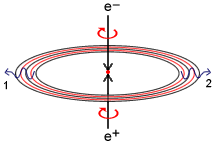Fig 10.3 Electron-positron annihilation.

In accordance with our model for the creation of a photon (see Light page), this rotational aether disturbance should give rise to an expanding photon ring, Fig 10.3.
Note that if our photon model is correct, the two photons moving in opposite directions that are normally detected with electron-positron annihilation experiments are not really two separate photons but the opposite ends of an expanding photon ring, Fig 10.3.
Because the 4D aether vortexes are spinning in opposite sense for the electron and positron, the two will simply cancel each other out when they meet, and will in fact be annihilated from existence. Leaving nothing but the aether rotational disturbance occuring prior to their collision.
Therefore, our model is consistent with the notion that it is the electromagnetic mass component that is converted into the energy of the emitted radiation.
What happens to the rest mass is not a problem in this model, the two particles or 4D vortexes simply cancel leaving no excess energy. There is in fact no electron rest mass as such. What are termed rest masses are really inertial masses which only show up upon acceleration, or the effective vortex masses as discussed in the Atoms&QM section.

The situation is a little different with proton-antiproton annihilations.
In our aether model the proton is considered to be a condensation of the aether particles into a solid like state, somewhat like a liquid to solid transition. The antiproton would simply be a proton which has an electron type vortex associated with it rather than the normal positive charge version.
The proton would therefore require some minimum energy input in order to re-expand back to the normal more rarified aether state, somewhat like a solid to liquid transition.
We postulate that the energy of the proton-antiproton collision would be sufficient to re-expand the two particles from a solid to the more rarified state of the surrounding aether. The two particles would cease to exist.
We believe it is this sudden re-expansion of the condensed aether particles that creates a disturbance of the surrounding aether giving rise to the more energetic photon emission than can be explained by the electromagnetic mass alone.
Unlike the electron, the proton does have a rest mass in the sense that there is a 'substance' there that is converted to energy. Experiments with proton-antiproton annihilations suggest that this conversion process follows the E=mc2 formula.
This in turn implies that there is some unifying principle behind all the 'mass' energy conversion processes that are described by the E=mc2 equation.
However, for reasons already discussed, we do not believe that special relativity is capable of providing that unifying principle.

#### Aetheric Doppler Shift

One of the other arguments that people use against the aether concept is the fact that the observed Doppler frequency shift for light agrees with the relativistic formula rather than the classical formula for waves carried by a medium.
We will show here that the Doppler shift for light is consistent with an aether model if we add time dilation.
The standard formulas for the frequency shift of any wave carried by a medium, such as sound carried by air, when the observer or source is moving relative to that medium are as follows.

Classic Doppler formula:source movingobserver moving

f   = observed frequency
fo = frequency when source and observer are both stationary relative to the medium
vs = velocity of source relative to the medium
vo = velocity of observer relative to the medium

Where vs and vo are assumed positive when the source or observer are receding and negative if approaching.

Relativistic Doppler formula:v  = relative velocity between source and observer (+ve receding, -ve approaching)
c  = speed of light

Let us now look more closely at what one would expect for the case of light being propagated through a medium.
Firstly the classical Doppler shifts should apply.
In addition, since we are postulating that atomic processes are slowed for an object moving relative to the aether (as discussed in the above section), we would expect that the frequency of the emitted radiation should also slow down for a moving source.
In other words, the frequency of emitted radiation should be reduced by the same relativistic factor that appears in the time dilation formula.
Therefore, we expect the complete Doppler shift formula for a light source moving with respect to the aether to be: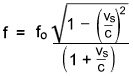or the mathematically equivalent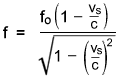Similarly, the internal processes for an observer will slow down if moving with respect to the aether. Hence, the moving observer will see a slightly higher frequency for the radiation relative to its own slowed down processes than would be the case for an observer stationary with respect to the aether.
Therefore the complete formula for the moving observer case would be:We can combine the above two formulas to give the Doppler shifts for the general case where both the source and observer are moving relative to the aether: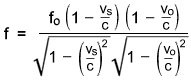f   = observed frequency
fo = frequency when source and observer are both stationary relative to the aether
vs = velocity of source relative to the aether (+ve receding, -ve approaching)
vo = velocity of observer relative to the aether (+ve receding, -ve approaching)
c  = speed of light

We will consider some consequences of this formula.
Firstly, we note that if either the source or the observer is at rest relative to the aether (ie vs or vo is zero), the above formula will be identical to the relativistic formula.
Since we are postulating that the aether is entrained by the earth, and is therefore stationary with respect to observers on the surface of the earth, any experiments where the observer is stationary on the earth's surface (vo = 0) and the source is moving (most experiments are of this type) will therefore show a Doppler shift equivalent to the relativistic formula.
The aetheric formula will differ from the relativistic one if both the observer and source move with respect to the aether. This would be one way the formula could be tested.

We note also that for extraterrestrial Doppler shift measurements, such as the red shift of stars, the aetheric formula will give values that depend on the speed of the source relative to its local aether, which generally will not be the same as the speed relative to the earthbound aether.
The difference between the two formulas will depend on how much movement there is between the earthbound aether and the aether local to the source.
This could help explain some of the anomalies that have been observed with astronomical observations, as discussed in the next section.

#### Big Bang and the Stellar Redshift

There are many discrepancies between observation of the red shift of stars and the theory of the expansion of the universe based on the Big Bang scenario. David Pratt  and Roger Rydin  have highlighted many of these , , , .
It seems that the more we look the more inconsistencies we find (see  for the latest problem) and we have to introduce ad-hoc factors, such as dark matter and energy, in order to save the standard theory. Dark matter theories themselves have recently been contradicted by experiments .
Apart from experimental discrepancies there are also conceptual and philosophical difficulties with the Big Bang idea.
Current theory has it that the universe is expanding from an origin point as a result of space itself expanding in all directions. However, for space to expand there needs to be something like aether particles to define the expansion. Without something to define space it becomes meaningless to talk about its expansion.
However, this in turn leads to other difficulties with the Big Bang scenario. If you run the expansion backwards what happens to these 'particles' at the singularity before the Big Bang explosion?
While solutions to these difficulties may be found in the future, it seems to this author that a much simpler explanation would be that the Big Bang never happened, the redshift can be explained in other ways.
According to standard theory, the amount that light from a star is redshifted is proportional to the velocity at which it is receding away from us due to the Doppler frequency shift.
The general picture is of a universe that resembles a balloon which is expanding from a central point of the Big Bang, with the result that all parts are moving away from each other. This is said to explain why there is a general redshift rather than a blueshift or a mixture of the two.

An alternative explanation for the redshift is the so called Tired Light model.
This assumes that the frequency shift of starlight is caused not by recession velocities but by the interaction of light with electrons that exist at low densities in interstellar space. The further away the source the more interactions light undergoes before reaching us and therefore the more redshifted it is.
See Lyndon Ashmore's work  for a more detailed discussion in support of this theory.

We would also like to present here an intriguing alternative theory for explaining the general redshift of stars that does not involve an expansion of the universe. The concept originates from master Hilarion (as channeled by Maurice Cooke), .
This possibility is based on the idea that our universe forms a sphere when viewed from the 4th dimension. That is, the thin (along the 4th dimension) membrane containing our physical 3D universe curves around to form an enormous 4D sphere (see the discussion in our Matter page).Fig 10.4  4D hypersphere model, giving an alternative explanation for the redshift
usually attributed to the expansion of the universe.

If light from distant objects reaches us by going through the body of the sphere rather than traveling along its surface, it will create an angle of incidence for the light as it hits 3D space at our location, Fig 10.4.
This is because the tangent to the surface of the 4D sphere (red lines in Fig 10.4) along which 3D objects vibrate to create light will be at a different angle in our part of the universe compared to that of a distant galaxy.
What we see when that light reaches us is not the original light wave but a projection of it onto the 3D plane, giving rise to longer wavelengths.
The further away the source is the greater the difference in angle and therefore the greater the redshift.

#### References

 Milan R. Pavlovic, “Einstein's Theory of Relativity - Scientific Theory or Illusion?", Milan Pavlovic
 G. Burniston Brown, “What is wrong with relativity?",  homepage.ntlworld.com/academ/whatswrongwithrelativity.html
 Marcus Coleman, "The Trouble with Relativity",  www.wbabin.net/physics/marcus.htm
 J. A. Hickli, "Reason Replaces Relativity",  www.relativitycalculator.com/pdfs/someeternalphysics.pdf
 G. O. Mueller, "95 Years of Criticism of the Special Theory of Relativity",  www.wbabin.net/science/mueller.pdf
 James DeMeo, "Dayton Miller's Ether-Drift Experiments",  www.orgonelab.org/miller.htm
 Yu. M. Galaev, “The Measuring of Ether-Drift Velocity and Kinematic Ether Viscosity within Optical Wave Bands", Spacetime &
Substance, Vol 3 (2002), No 5 (15), pp. 207-224  www.mountainman.com.au/aether_6.htm
 Yahoo News, "Light can break it's own speed limit",  Yahoo News
 Eugene Podkletnov, "Superconductors and Gravity-Modification",  www.pureenergysystems.com
 Anti-Relativity website, www.anti-relativity.com
 Webster Kehr, "The Detection of Ether",  www.teslaphysics.com
 Charles R. Morton, "Velocity Alters Electric Field", www.amasci.com/freenrg/morton1.html
 David Wilcock, “Divine Cosmos",  www.divinecosmos.com
 H. E. Ives, 1952, J. Opt. Soc. Amer., 42, 540—3
 Milan R. Pavlovic, “Mass and Energy", Ch. 23, Milan Pavlovic
 Milan R. Pavlovic, “Antimatter and the Annihilation of Matter and Antimatter Do Not Exist", Ch. 26,  Milan Pavlovic
 David Pratt, "Beyond the Big Bang", davidpratt.info/cosmo.htm
 Roger A. Rydin, "Big Bang? Public Forum",  home.earthlink.net/~rarydin
 "An Open Letter to the Scientific Community", New Scientist, May 22 2004
 Donald E. Scott, "Redshift", www.electric-cosmos.org/arp.htm
 Meta Research, "The Top 30 Problems with the Big Bang", see  www.metaresearch.org/cosmology/BB-top-30.asp
 Paul Marmet, "Newton Physics", www.newtonphysics.on.ca
 Eric J Lerner, "The Big Bang Never Happened", www.bigbangneverhappened.org

 Physorg.com, "Big Bang's Afterglow Fails an Intergalactic Shadow Test",   www.physorg.com/news76314500.html
 Lyndon Ashmore, "Tired Light Model", www.lyndonashmore.com
 Hilarion (Maurice B. Cooke), “The Nature of Reality”, Marcus Books, PO Box 327, Queensville, Ontario, Canada L0G 1R0, 1990
 ESO, "Serious Blow to Dark Matter Theories?", www.eso.org/public/news/eso1217/#3
 Juan Yin et. al., "Bounding the speed of `spooky action at a distance'", MIT Technology Review